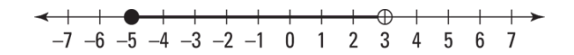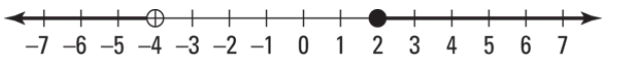# Accuplacer Arithmetic Practice Test 2023 [NextGen]

Accuplacer Arithmetic Practice Test  2023: Try our Accuplacer NextGen Arithmetic Practice Questions Answers. You can also download printable copy of PDF file. This Accuplacer Arithmetic Practice Test includes Working with whole numbers, Solving problems using fractions, decimals, and percents, and Setting up and solving word problems.

This Next-generation Accuplacer Arithmetic Practice Test  2022 describes the skills necessary to do well on the ACCUPLACER Arithmetic Test. In this chapter, you put these skills to work with practice problems.

## Accuplacer Arithmetic Practice Test 2023 [NextGen]

 Name of the Test Accuplacer Practice Test Administrated by College Board Total questions 30 Question format multiple-choice questions Test subjects Arithmetic

Whole numbers practice test

Q1. What is 8,765,432 rounded to the nearest thousand?

• (A). 8,765,000
• (B). 8,432,000
• (C). 8,700,000
• (D). 8,600,000

Q2. If you multiply 786 × 2971, which of the following best approximates the answer?

• (A) 140,000
• (B) 240,000
• (C) 1,400,000
• (D) 2,400,000

Q3. What is the value of 15 − 4² ÷ (32 − 8 × 3)

• (A). 130
• (B). 3
• (C). 31
• (D). 13

Q4. Which of the following inequalities is equivalent to the one shown in the figure?• (A) – 5 ≤ x < 3
• (B) – 5 < x ≤ 3
• (C) x ≤ – 5 or x > 3
• (D) x < – 5 or x ≥ 3

Q5. Which of the following would be the best estimation for 451327 ÷ 8946

• A) 5
• (B) 50
• (C) 500
• (D) 5000

Q6. Evaluate (17 – 2³) × (6 + 10 ÷ 2)

• (A). 9
• (B). 39
• (C). 49
• (D). 99

Q7. Which of these inequalities expresses the solution set for the number line shown in the following figure?• (A) −4 ≤ x < 2
• (B) −4 < x ≤ 2
• (C) x ≤ −4 or x > 2
• (D) x < −4 or x ≥ 2

Q8. What is 939,594 rounded to the nearest hundred?

• (A) 939,600
• (B) 939,500
• (C) 930,600
• (D) 939,700

Fractions number practice test

Q9. \frac79 + \frac59 = ?

• (A) \frac19
• (B) 1\frac19
• (C) \frac13
• (D) 1 \frac13

Q10.   \frac{11}{27} ÷ \frac{22}{23} = ?

• (A)   \frac{32}{45}
• (B)   \frac{2}{5}
• (C)   \frac{23}{3}
• (D)   \frac{23}{54}

Q11.   2\frac{1}{5} × 3 \frac{3}{4} = ?

• (A)   2\frac{1}{4}
• (B)   1\frac{8}{3}
• (C)   8\frac{1}{4}
• (D)   4\frac{1}{8}

Q12.   \frac{2}{7} − \frac{1}{3} = ?

• (A) \frac{9}{19}
• (B)   \frac{3}{91}
• (C)   1\frac{1}{9}
• (D)   \frac{19}{91}

Decimals number practice test

Q13. 0.835 + 1.09 =?

• (A) 0.925
• (B) 1.925
• (C) 2.925
• (D) 3.925

Q14. 4.4 + 82.39 + 0.027 = ?

• (A) 68.717
• (B) 96.617
• (C) 16.617
• (D) 86.817

Q15. 42.563 − 8.05 =?

• (A)  34.513
• (B)  33.513
• (C) 34.613
• (D) 33.513

Q16. 0.0748 × 3.6 =?

• (A) 0.36928
• (B) 0.16928
• (C) 0.26928
• (D) 1.26928

Q17. 32.175 ÷ 1.8 = ?

• (A) 16.875
• (B) 15.875
• (C) 17.875
• (D) 19.875

Percent Practice Test

Q18. Jacqui took her friend, Bethany, out to lunch for her birthday. When the check arrived, it was for $45.00. If Jacqui included an 18% tip for the server, what did she pay in total for the lunch? • (A)$3.10
• (B) $5.10 • (C)$35.10
• (D) $53.10 Show Answers Answer: option D Q19. By changing phone plans, Blair received a 30% discount on a phone that normally sells for$780. How much did she pay for the phone?

• (A) $346 • (B)$446
• (C) $546 • (D)$646

Q20. Drew invested $6,600 in a stock that lost 15% of its value before Drew sold it. How much was the stock worth at the time of sale? • (A) 77.85 • (B) 75.75 • (C) 87.87 • (D) 87.75 Show Answers Answer: option D Q21. What percent of 120 is 72? • (A) 40% • (B) 45% • (C) 60% • (D) 65% Show Answers Answer: option D Fraction, decimal, percent conversion practice test Q22. Convert the decimal 0.72 to a fraction. • (A) \frac{18}{25} • (B) \frac{1}{25} • (C) \frac{9}{25} • (D) \frac{3}{25} Show Answers Answer: option A Q23. Write the decimal 0.375 as a percent. • (A) 7.5% • (B) 17.5% • (C) 27.5% • (D) 37.5% Show Answers Answer: option D Q24. What is the value of 188.1% expressed as a decimal? • (A) 0.302 • (B) 0.102 • (C) 1.302 • (D) 0.202 Show Answers Answer: option A Comparing values practice test Q25. Which of the following decimals is the greatest? • (A) 0.1811 • (B) 0.088 • (C) 0.0188 • (D) 0.801 Show Answers Answer: option D Q26. Which of these decimals is the greatest? • (A) 0.3054 • (B) 0.306 • (C) 0.32 • (D) 0.321 Show Answers Answer: option D Word problem practice test Q27. Anjelika spent$35.75 on a sweater and $56.99 on a pair of shoes. How much more did the shoes cost than the sweater? • (A)$21.24
• (B) $12.21 • (C)$24.21
• (D) $12.24 Show Answers Answer: option A Q28. Eric is supervising a group of 57 children at a summer camp. He wants to rent a set of rowboats, each of which will hold a maximum of six children. What is the minimum number of rowboats that Eric will need to rent to make sure that every child is included? • (A) 1 rowboats • (B) 5 rowboats • (C) 10 rowboats • (D) 15 rowboats Show Answers Answer: option C Q29. As an incentive for her sales staff of 12 people, Shondra allocated$7500 for a quarterly bonus, which she promised to split evenly among all salespeople who made their quotas. If all 12 people succeeded, how much money did each person receive?

• (A) $25 • (B)$125
• (C) $325 • (D)$625